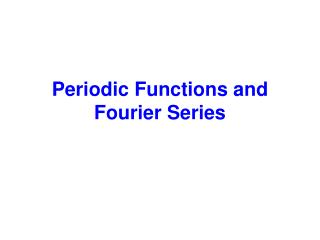# Periodic Functions and Fourier Series - PowerPoint PPT PresentationDownload PresentationPeriodic Functions and Fourier Series

Periodic Functions and Fourier SeriesDownload Presentation## Periodic Functions and Fourier Series

- - - - - - - - - - - - - - - - - - - - - - - - - - - E N D - - - - - - - - - - - - - - - - - - - - - - - - - - -
##### Presentation Transcript

1. Periodic Functions and Fourier Series

2. A function is periodic if it is defined for all real such that Periodic Functions and if there is some positive number, .

3. be a periodic function with period Fourier Series The function can be represented by a trigonometric series as:

4. and . We want to determine the coefficients, Let us first remember some useful integrations.

5. for all values of m.

6. Determine to Integrate both sides of (1) from

7. is the average (dc) value of the function, .

8. to instead. You may integrate both sides of (1) from It is alright as long as the integration is performed over one period.

9. Determine Multiply (1) by to and then Integrate both sides from

10. First term, Second term, Let us do the integration on the right-hand-side one term at a time.

11. Second term, Third term,

12. Therefore,

13. Determine Multiply (1) by to and then Integrate both sides from

14. First term, Second term, Let us do the integration on the right-hand-side one term at a time.

15. Second term, Third term,

16. Therefore,

17. The coefficients are:

18. We can write n in place of m:

19. The integrations can be performed from to instead.

20. Therefore, the corresponding Fourier series is In writing the Fourier series we may not be able to consider infinite number of terms for practical reasons. The question therefore, is – how many terms to consider?

21. 1.5 1 0.5 f ( q ) 0 0.5 1 1.5 q When we consider 4 terms as shown in the previous slide, the function looks like the following.

22. 1.5 1 0.5 f ( q ) 0 0.5 1 1.5 q When we consider 6 terms, the function looks like the following.

23. 1.5 1 0.5 f ( q ) 0 0.5 1 1.5 q When we consider 8 terms, the function looks like the following.

24. 1.5 1 0.5 f ( q ) 0 0.5 1 1.5 q When we consider 12 terms, the function looks like the following.

25. 1.5 1 0.5 0 0.5 1 1.5 q The red curve was drawn with 12 terms and the blue curve was drawn with 4 terms.

26. 1.5 1 0.5 0 0.5 1 1.5 0 2 4 6 8 10 q The red curve was drawn with 12 terms and the blue curve was drawn with 4 terms.

27. 1.5 1 0.5 0 0.5 1 1.5 0 2 4 6 8 10 q The red curve was drawn with 20 terms and the blue curve was drawn with 4 terms.

28. Even and Odd Functions (We are not talking about even or odd numbers.)

29. Mathematically speaking - Even Functions The value of the function would be the same when we walk equal distances along the X-axis in opposite directions.

30. Mathematically speaking - Odd Functions The value of the function would change its sign but with the same magnitude when we walk equal distances along the X-axis in opposite directions.

31. 5 0 5 10 0 10 q Even functions can solely be represented by cosine waves because, cosine waves are even functions. A sum of even functions is another even function.

32. 5 0 5 10 0 10 q Odd functions can solely be represented by sine waves because, sine waves are odd functions. A sum of odd functions is another odd function.

33. The Fourier series of an even function is expressed in terms of a cosine series. The Fourier series of an odd function is expressed in terms of a sine series.